# Binary Tree | Types of Binary Trees

## Tree Data Structure-

Before you go through this article, make sure that you have gone through the previous article on Tree Data Structure.

We have discussed-

• Tree is a non-linear data structure.
• In a tree data structure, a node can have any number of child nodes.

## Binary Tree-

 Binary tree is a special tree data structure in which each node can have at most 2 children. Thus, in a binary tree, Each node has either 0 child or 1 child or 2 children.

## Example-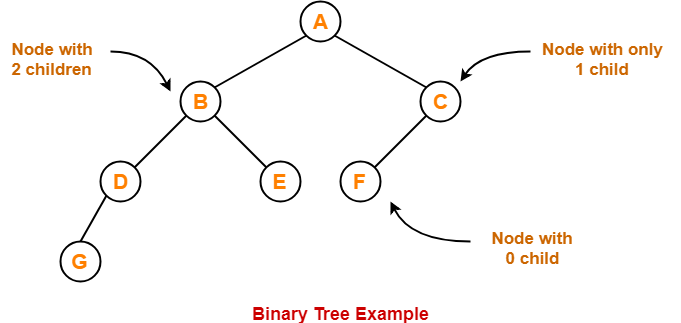## Unlabeled Binary Tree-

 A binary tree is unlabeled if its nodes are not assigned any label.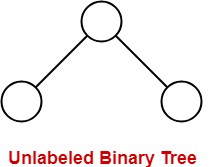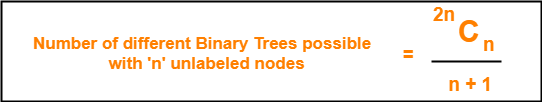## Example-

Consider we want to draw all the binary trees possible with 3 unlabeled nodes.

Using the above formula, we have-

Number of binary trees possible with 3 unlabeled nodes

= 2 x 3C3 / (3 + 1)

= 6C3 / 4

= 5

Thus,

• With 3 unlabeled nodes, 5 unlabeled binary trees are possible.
• These unlabeled binary trees are as follows-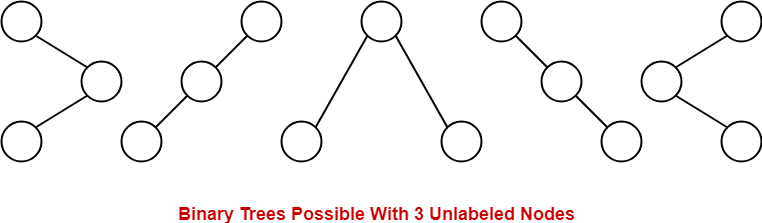## Labeled Binary Tree-

 A binary tree is labeled if all its nodes are assigned a label.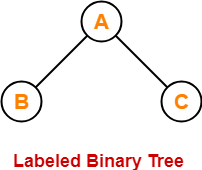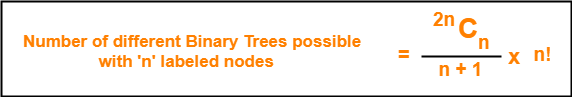## Example-

Consider we want to draw all the binary trees possible with 3 labeled nodes.

Using the above formula, we have-

Number of binary trees possible with 3 labeled nodes

= { 2 x 3C3 / (3 + 1) } x 3!

= { 6C3 / 4 } x 6

= 5 x 6

= 30

Thus,

• With 3 labeled nodes, 30 labeled binary trees are possible.
• Each unlabeled structure gives rise to 3! = 6 different labeled structures.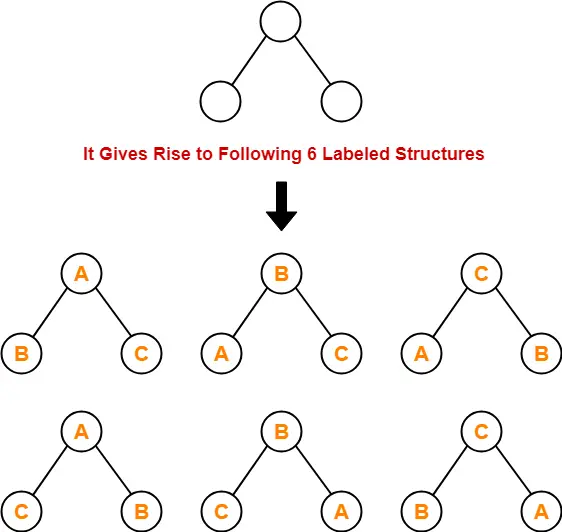Similarly,

• Every other unlabeled structure gives rise to 6 different labeled structures.
• Thus, in total 30 different labeled binary trees are possible.

## Types of Binary Trees-

Binary trees can be of the following types-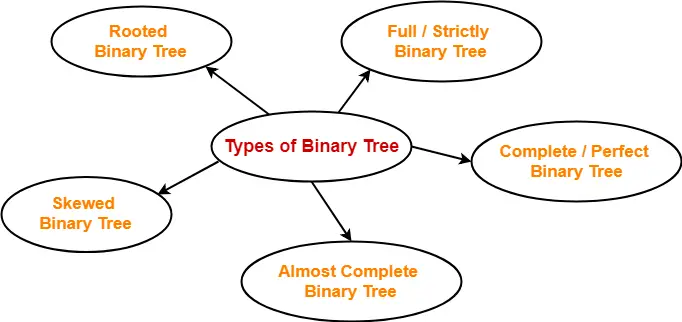1. Rooted Binary Tree
2. Full / Strictly Binary Tree
3. Complete / Perfect Binary Tree
4. Almost Complete Binary Tree
5. Skewed Binary Tree

## 1. Rooted Binary Tree-

A rooted binary tree is a binary tree that satisfies the following 2 properties-

• It has a root node.
• Each node has at most 2 children.

### Example-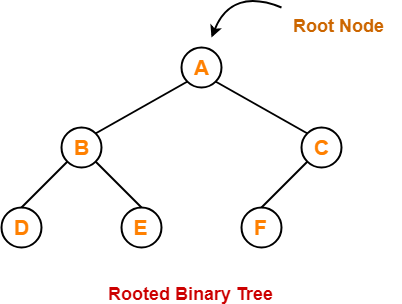## 2. Full / Strictly Binary Tree-

• A binary tree in which every node has either 0 or 2 children is called as a Full binary tree.
• Full binary tree is also called as Strictly binary tree.

### Example-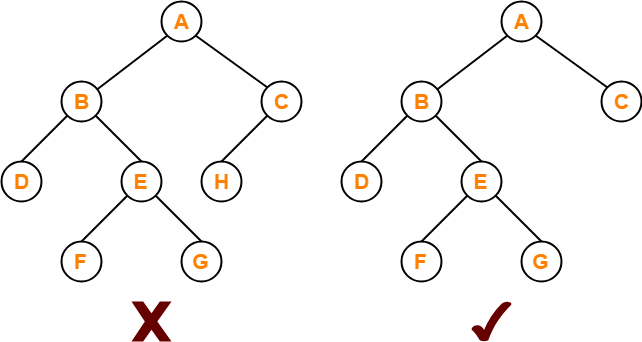Here,

• First binary tree is not a full binary tree.
• This is because node C has only 1 child.

## 3. Complete / Perfect Binary Tree-

A complete binary tree is a binary tree that satisfies the following 2 properties-

• Every internal node has exactly 2 children.
• All the leaf nodes are at the same level.

Complete binary tree is also called as Perfect binary tree.

### Example-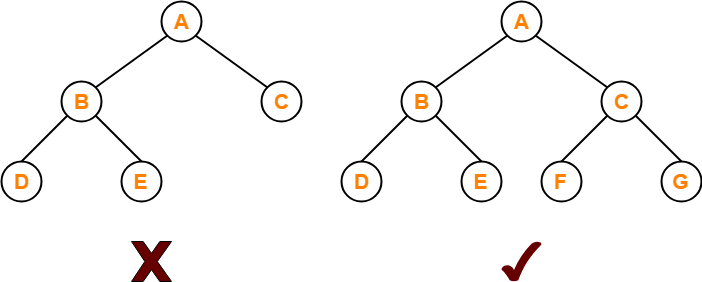Here,

• First binary tree is not a complete binary tree.
• This is because all the leaf nodes are not at the same level.

## 4. Almost Complete Binary Tree-

An almost complete binary tree is a binary tree that satisfies the following 2 properties-

• All the levels are completely filled except possibly the last level.
• The last level must be strictly filled from left to right.

### Example-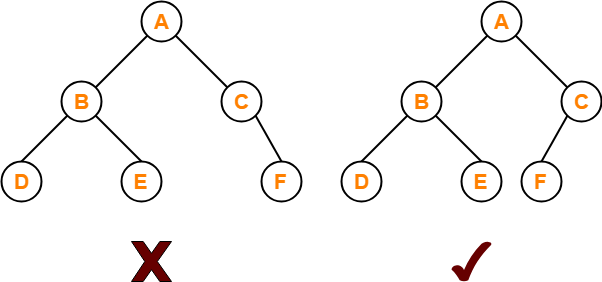Here,

• First binary tree is not an almost complete binary tree.
• This is because the last level is not filled from left to right.

## 5. Skewed Binary Tree-

A skewed binary tree is a binary tree that satisfies the following 2 properties-

• All the nodes except one node has one and only one child.
• The remaining node has no child.

OR

A skewed binary tree is a binary tree of n nodes such that its depth is (n-1).

### Example-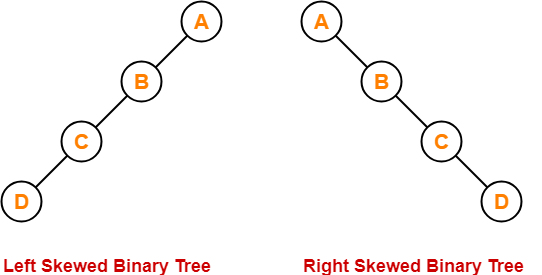To gain better understanding about Binary Tree and its types-

Watch this Video LectureNext Article- Binary Tree Properties

Get more notes and other study material of Data Structures.

Watch video lectures by visiting our YouTube channel LearnVidFun.

SummaryArticle Name
Binary Tree | Types of Binary Trees
Description
Binary tree is a special tree data structure. Types of Trees in Data Structure- Perfect or Complete Binary Tree, Full or Strictly Binary Tree, Almost Complete Binary Tree, Skew Binary Tree, Rooted Binary Tree, Balance Binary Tree.
Author
Publisher Name
Gate Vidyalay
Publisher Logo